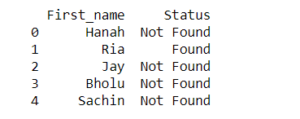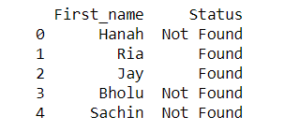# Ways to apply an if condition in Pandas DataFrame

Let’s discuss the different ways of applying If condition to a data frame in pandas.

1) Applying IF condition on Numbers
Let us create a Pandas DataFrame that has 5 numbers (say from 51 to 55). Let us apply IF conditions for the following situation. If the particular number is equal or lower than 53, then assign the value of ‘True’. Otherwise, if the number is greater than 53, then assign the value of ‘False’

Syntax:

df.loc[df[‘column name’] condition, ‘new column name’] = ‘value if condition is met’

Example:

 `from` `pandas ``import` `DataFrame ` ` `  `numbers ``=` `{``'mynumbers'``: [``51``, ``52``, ``53``, ``54``, ``55``]} ` `df ``=` `DataFrame(numbers, columns ``=``[``'mynumbers'``]) ` ` `  `df.loc[df[``'mynumbers'``] <``=` `53``, ``'<= 53'``] ``=` `'True'`  `df.loc[df[``'mynumbers'``] > ``53``, ``'<= 53'``] ``=` `'False'`  ` `  `df `

Output:2) Applying IF condition with lambda
Let us create a Pandas DataFrame that has 5 numbers (say from 51 to 55). Let us apply IF conditions for the following situation. If the particular number is equal or lower than 53, then assign the value of ‘True’. Otherwise, if the number is greater than 53, then assign the value of ‘False’.

Syntax:

df[‘new column name’] = df[‘column name’].apply(lambda x: ‘value if condition is met’ if x condition else ‘value if condition is not met’)

Example:

 `from` `pandas ``import` `DataFrame ` ` `  `numbers ``=` `{``'mynumbers'``: [``51``, ``52``, ``53``, ``54``, ``55``]} ` `df ``=` `DataFrame(numbers, columns ``=``[``'mynumbers'``]) ` ` `  `df[``'<= 53'``] ``=` `df[``'mynumbers'``].``apply``(``lambda` `x: ``'True'` `if` `x <``=` `53` `else` `'False'``) ` ` `  `print` `(df) `

Output:3) Applying IF condition on strings
We will deal with the DataFrame that contains only strings with 5 names: Hanah, Ria, Jay, Bholu, Sachin. The conditions are: If the name is equal to ‘Ria, ’ then assign the value of ‘Found’. Otherwise, if the name is not ‘Ria, ’ then assign the value of ‘Not Found’.

Example:

 `from` `pandas ``import` `DataFrame ` ` `  `names ``=` `{``'First_name'``: [``'Hanah'``, ``'Ria'``, ``'Jay'``, ``'Bholu'``, ``'Sachin'``]} ` `df ``=` `DataFrame(names, columns ``=``[``'First_name'``]) ` ` `  `df.loc[df[``'First_name'``] ``=``=` `'Ria'``, ``'Status'``] ``=` `'Found'`   `df.loc[df[``'First_name'``] !``=` `'Ria'``, ``'Status'``] ``=` `'Not Found'`  ` `  `print` `(df) `

Output:4) Applying IF condition on strings using lambada
We will deal with the DataFrame that contains only strings with 5 names: Hanah, Ria, Jay, Bholu, Sachin. The conditions are: If the name is equal to ‘Ria, ’ then assign the value of ‘Found’. Otherwise, if the name is not ‘Ria, ’ then assign the value of ‘Not Found’. But this time we will deal with it using lambdas.

Example

 `from` `pandas ``import` `DataFrame ` ` `  `names ``=` `{``'First_name'``: [``'Hanah'``, ``'Ria'``, ``'Jay'``, ``'Bholu'``, ``'Sachin'``]} ` `df ``=` `DataFrame(names, columns ``=``[``'First_name'``]) ` ` `  `df[``'Status'``] ``=` `df[``'First_name'``].``apply``(``lambda` `x: ``'Found'` `if` `x ``=``=` `'Ria'` `else` `'Not Found'``) ` ` `  `print` `(df) `

Output:5) Applying IF condition with OR
We will deal with the DataFrame that contains only strings with 5 names: Hanah, Ria, Jay, Bholu, Sachin. The conditions are: If the name is equal to “Ria”, or “Jay” then assign the value of ‘Found’. Otherwise, if the name is not “Ria” or “Jay” then assign the value of ‘Not Found’.

Example

 `from` `pandas ``import` `DataFrame ` ` `  `names ``=` `{``'First_name'``: [``'Hanah'``, ``'Ria'``, ``'Jay'``, ``'Bholu'``, ``'Sachin'``]} ` `df ``=` `DataFrame(names, columns ``=``[``'First_name'``]) ` ` `  `df.loc[(df[``'First_name'``] ``=``=` `'Ria'``) | (df[``'First_name'``] ``=``=` `'Jay'``), ``'Status'``] ``=` `'Found'`   `df.loc[(df[``'First_name'``] !``=` `'Ria'``) & (df[``'First_name'``] !``=` `'Jay'``), ``'Status'``] ``=` `'Not Found'`   ` `  `print` `(df) `

Output:My Personal Notes arrow_drop_upCheck out this Author's contributed articles.

If you like GeeksforGeeks and would like to contribute, you can also write an article using contribute.geeksforgeeks.org or mail your article to contribute@geeksforgeeks.org. See your article appearing on the GeeksforGeeks main page and help other Geeks.

Please Improve this article if you find anything incorrect by clicking on the "Improve Article" button below.

Article Tags :

Be the First to upvote.

Please write to us at contribute@geeksforgeeks.org to report any issue with the above content.